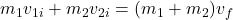## Car A of mass 1200kg traveling at 10m/s , collided in too the back of the car B, which is stationary. Following the collision,. The two cars

Question

Car A of mass 1200kg traveling at 10m/s , collided in too the back of the car B, which is stationary. Following the collision,. The two cars stick together and travel at 6m/s. What is the mass of a car B?

in progress 0
6 months 2021-08-04T13:08:52+00:00 2 Answers 0 views 0

Explanation:

Caraofmass1200kg

Car B has a mass of 800 kg.

General Formulas and Concepts:

Momentum

Law of Conservation of Momentum:Explanation:

Step 1: Define

Identify variables

[Given] m₁ = 1200 kg

[Given] v₁i = 10 m/s

[Solve] m₂

[Given] v₂i = 0 m/s

[Given] vf = 6 m/s

Step 2: Solve for m₂

1. Substitute in variables [Law of Conservation of Momentum]:                       (1200 kg)(10 m/s) + m₂(0 m/s) = (1200 kg + m₂)(6 m/s)
2. Multiply:                                                                                                             12000 kg · m/s = (1200 kg + m₂)(6 m/s)
3. Isolate m₂ term:                                                                                                2000 kg = 1200 kg + m₂
4. Isolate m₂:                                                                                                         800 kg = m₂